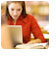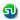### TANCET 2008 - Past year paper: Word Problems, Framing equations: Ascent Education TANCET MBA Classes

 Home TANCET Classes GMAT Coaching Online GMAT Course CAT Classes TANCET Study Material
 TANCET '18 Classroom ProgramWeekend and weekday classes for TANCET MBA @ Chennai. At Nungambakkam and Velachery.Other Courses TANCET Correspondence Course CAT Classes & Courses GMAT Classes Chennai Online GMAT Course GRE Classes Chennai CBSE Math Online Tuition SAT Classes Other Links Ascent TANCET Toppers Testimonials CAT, TANCET Questions Careers @ Ascent Contact Us +91 44 4500 8484 +91 96000 48484 ascent@ascenteducation.com Postal Address Facebook / Twitter / Blog / Videos
You are here: Home  »  XAT, TANCET Prep Questions »  Percents, Fractions »   TANCET 2008 Question 8

# TANCET 2008 Quant Question 8 : Framing Expressions

## Question

It costs Rs. x each to make the first thousand copies of a compact disk and Rs. y to make each subsequent copy. If z is greater than 1,000, how many Rupees will it cost to make z copies of the compact disk?
1. 1,000 x + yz
2. zx - zy
3. 1,000 (z - x) + xy
4. 1,000 (z - y) + xz
5. 1,000 (x - y) + yz
Correct Answer: 1,000 (x - y) + yz. Choice (5)

We need to find the total cost to make z copies, z > 1000.

The first 1000 copies will cost Rs.x each. Or the total cost of first 1000 copies = Rs.1000x
The remaining z - 1000 copies will cost Rs.y each.
Or the cost of the z - 1000 = Rs.(z - 1000)y

Therefore, total cost = 1000x + zy - 1000y
= 1000(x - y) + yz

Level of difficulty : Easy

## More Questions on Linear, Quadratic and Cubic Equations - Algebra

 5. 6. 7.

## CAT, XAT, TANCET Practice Questions and Answers : Listed Topicwise

 Number Theory Permutation Combination Probability Inequalities Geometry Mensuration Trigonometry Coordinate Geometry Percentages Profit Loss Ratio Proportion Mixtures Alligation Speed Time Distance Pipes Cisterns Interest Races Average, Mean AP, GP, HP Set Theory Clocks Calendars Algebra Function English Grammar General Awareness Data sufficiency TANCET Papers XAT PapersAdd to del.icio.usStumble Itdigg this